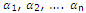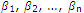# Describe the transformations of equations

Publish On: 2019-02-24

Total Post: 559

# Mjay Jollay

Total Post: 0

## ANS: Describe the transformations of equations

If an equation is given, then it is possible for us to transform it into another such that the rots of the new equation have certain assigned relations with the roots of the given equation. The new equation is called transformed equation. The transformation of an equation sometimes facilitates in determining the roots of the given equation by solving the transformed equation, which is easier to solve. In this, section, we shall discuss the most important transformations. In the following article we shall assume thatare the roots of the given equation ƒ(x) = 0 andare the roots of the required equation.